amortization.com Ltd.
Burlington, Ontario

905-639-0374
905-407-7988

info@amortization.comModified 0% Loan

Calculating the APR of a modified 0% loan using a 12% annual interest rate and a two year amortization period is shown below. The 12% interest rate was chosen so that the monthly interest charges are easier to view. The two year amortization period was selected so the complete schedule could be shown on the screen captures.

A 24 monthly payment, \$18,000 loan has the first six months at zero interest and the remaining 18 months at 12%. Monthly was selected.

QUESTION: What is the APR because of the first six months at zero interest?

1.. The first step is to generate a normal amortization schedule. The interest rate will be modified inside the amortization schedule. NOTE: The interest portion of the first payment is \$180 which is 1% per month. It is easy to visualize moving the decimal place two spaces to the left to arrive at \$180 interest cost for a loan of \$18,000. 0.01 x 18,000 = 180

(Screenshot 1)
*Note* When viewing the screenshot for this example, look for this symbol in the bottom right hand corner to expand the image to fullscreen: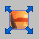2.. This next step is not necessary in solving the problem, however it is shown in order to see the connection between the Present Value of a loan the the Future Value of a loan and how they relate to an amortization schedule. The Principal amount of \$18,000 is really the Present Value and the Future Value is \$22,855.20. The Future Value of \$22,855.20 is what monthly payments (actually monthly deposits into an account) of \$847.32 would accumulate to after 24 months growing at an annual stated rate of 12% using monthly compounding. The effective interest rate (EIR) is 12.6825% at four decimal places. Notice that the Present Value Future Value calculation calculates the effective interest rate (EIR) if the period chosen is a year, as it should (by definition). The negative amortization schedule generated in this step 2 was easily accomplished by changing the first payment in the schedule to zero, highlighting the next 23 payments (with the mouse or the shift+down arrow) and then copying it down to payment 24 using the Ctrl+V option.

(Screenshot 2)

3.. This is the step where the actual calculation is done. Take the normal amortization schedule and insert an extra payment at the begining of the schedule using the yellow icon in the toolbar. This allows you to make the interest charge zero for the first payment. February 15th 2003 becomes payment 0 and the balance or Principal on that date is \$18,000. The green cell is coloured to bring to your attention that the interest for a given period of time is shown in the prior month. To make zero interest in effect for the period (actually the first payment) Feb 15th to March 15th, you type in zero in the green cell. Payment 25 (which is really payment 24) is for all practical purposes has a zero balance (6 cents) and is coloured light blue to bring it to your attention. It is often impossible to get balances to agree to the penny because of rounding off.

(Screenshot 3)

4.. You can then type in zero for the next five periods and the resulting schedule will appear as shown. Payment 7 is really the sixth payment as an extra payment line was inserted into the schedule.

NOTE!!! Because of the first six months at zero percent the the borrower pays six payments of 847.32 at zero interest and 16 payments of 847.32 at 12% and the last payment of \$527.42 at 12%. There is one less payment now because of the zero interest charge in the first six payments.

(Screenshot 4)

5.. You are almost there. Now a negative amortization schedule needs to be done, in order to arrive at the EIR for this new schedule, by changing the first payment in the schedule to zero, highlighting the next 23 payments (with the mouse or the shift+down arrow) and then copying it down to payment 24 using the Ctrl+V option.

(Screenshot 5)

6.. The Future Value of this cash flow is \$21,317.46 and the EIR is now calculated as shown to be 8.8257%.

(Screenshot 6)

If this was a Canadian loan the 8.8257% would be the answer and it would be called the total cost of borrowing because the Canadian rules and regulations call for the new EIR to be quoted.

In the USA the APR is really the new stated annual interest rate thus we need to go one step further for US regulations. We need to calculate the annual interest rate that gives an EIR of 8.8257% and that is the true APR and the answer to this question.

Using the calculator play around by typing in an annual interest rate that gives an EIR (to the right) of 8.83% to two decimal places. using this method the answer is an APR of 8.49%

(Screenshot 7)

The mathematically inclined may want to use the formula;

EIR = {(1+R/12)^12}-1 for monthly compounding, where R is the annual interest rate (AIR). The symbol "^" is the exponent symbol and means (1+R/12) is multiplied by itself 12 times.

Rearranged the formula becomes;

R= 12{((1+EIR)^(1/12))-1}

substituting the values of EIR=8.8257 the formula calculation gives 8.4876% which is 8.49 to two decimal places.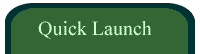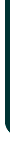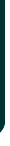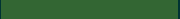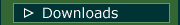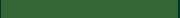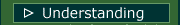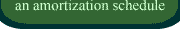VIDEOSamortizationdotcom Mortgage Calculator for iPhone

Introduction to Canadian and American Mortgages

Seminar on prepaying principal (Part A)

Seminar on prepaying principal (Part B)

Global TV Interview regarding 40 Year MortgagesLook for this logo on the Apple Store!

 < Go Back